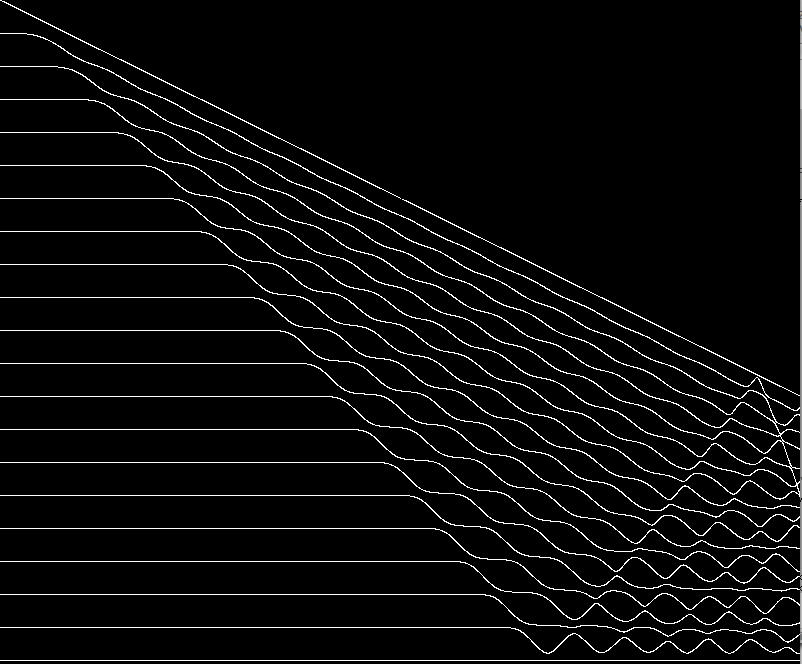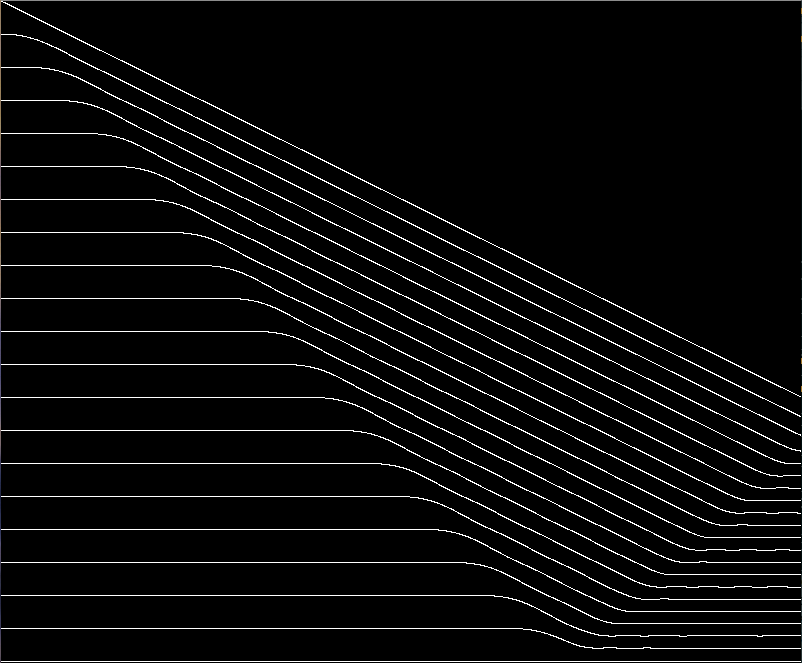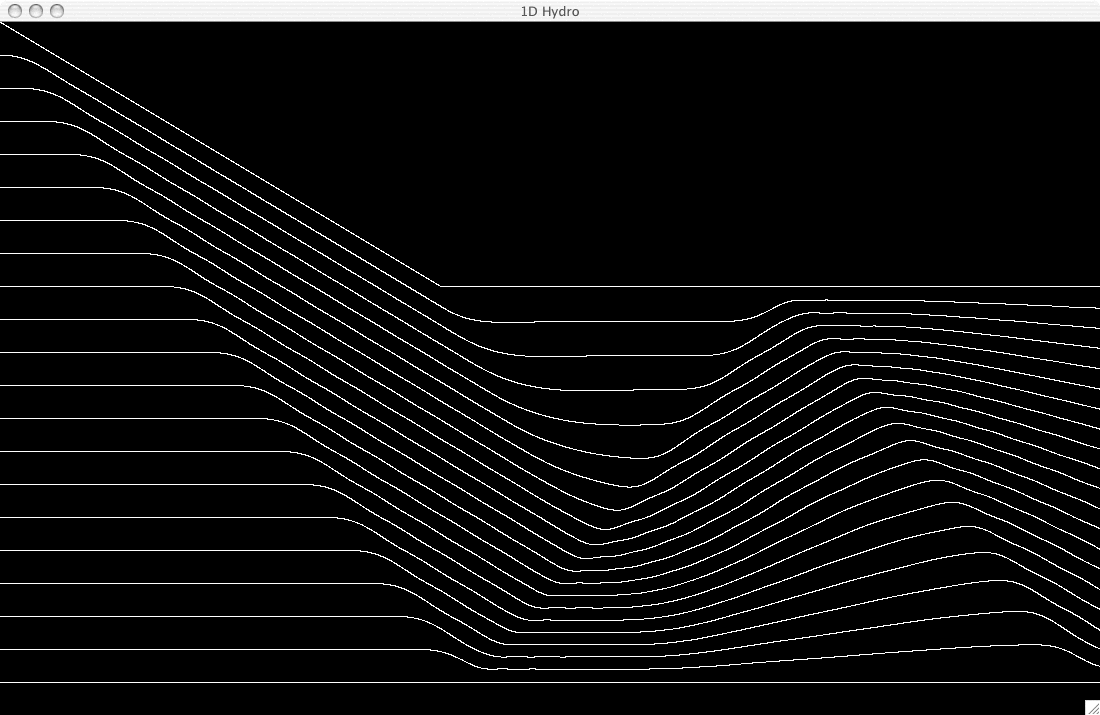This is about the simplest possible Lagrangian hydrodynamics program. It is one dimensional. It is for a perfect gas. It has equal mass zones. The Equation of State is P = T/V. The program is set up to do a simple shock wave calculation in which a piston compresses a body of gas initially at rest. The other end of the gas column is fixed and reflects the shock.

Zone numbering goes down. The dynamic variables per zone are collected into a structure m[i]:

• T = Temperature, m[i].T for zone i,
• x = Position of top end of zone, m[i].x for zone i,
• v = Velocity of top end of zone, m[i].v for zone i
In the following differences from time step to time step are expressed with Δ while differences from zone to zone with δ. With V = volume = δx and t = time, the calculation proceeds as follows:
• Δx = vΔt
• Δv = δPΔt
• V = δx
• P = T/V
• ΔT = PΔV

This is the first version of the code which produces the following picture: (Time is horizontal and displacement is vertical.)Notice the standing sound waves following the shock. This may be explained by noting that the difference equations compress the zones adiabatically whereas a real shock wave increases the entropy. Von Neumann proposed a Q = ρ(Δv)2 term sometimes called artificial viscosity. Q = 0 when Δv > 0. It augments pressure in zones which are compressing and thus increases the PΔV work to (P+Q)ΔV. This extra work on the zone increases the entropy and also damps the sound waves which otherwise attempt valiantly to model the kinetic energy that should have gone into heat. This code includes Von Neumann’s Q and produces the following:Von Neumann & Richtmeyer wrote a small book which I cannot now locate that covered these issues together with stability of calculations. This covered the Q idea.

Next we make the piston stop part way thru and this introduces a rarefaction wave. A rarefaction, which is much more complex, cannot be computed by hand as can a shock wave. A rarefaction wave disperses whereas a shock wave does not.Some history of these and related ideas

The first picture was made by these shell commands:

```javac 1Dnq.java
java Main```